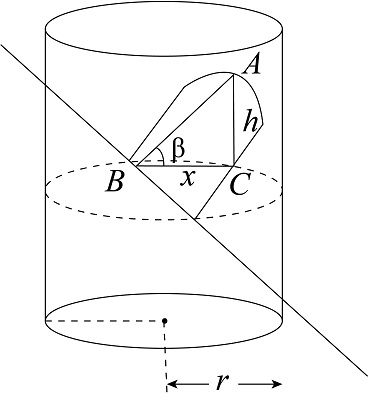# A wedge is cut from a right circular cylinder of radius r by two planes, one perpendicular to...

## Question:

A wedge is cut from a right circular cylinder of radius {eq}r {/eq} by two planes, one perpendicular to the axis of the cylinder and the other making an angle {eq}\beta {/eq} with the first. Find the volume of the wedge by slicing perpendicular to the y-axis.

## Definite Integration:

The definite integral is used to find the volume of a three-dimensional solid of revolution that results from revolving a two-dimensional region about a particular axis by taking cuts opposite to the axis of revolution.

A wedge is cut from a right circular cylinder of radius r by two planes is shown below.The area of triangle ABC is

{eq}A = \dfrac{1}{2}hx ......(i) {/eq}

Using the geometry in triangle ABC.

{eq}\begin{align*} \tan \beta &= \dfrac{h}{x}\\ h &= x\tan \beta \end{align*} {/eq}

Here, the height of triangle is h.

Substitute all the values in the above equation (i).

{eq}\begin{align*} A &= \dfrac{1}{2}\left( {x\tan \beta } \right)x\\ A &= \dfrac{1}{2}{x^2}\tan \beta.........(ii) \end{align*} {/eq}

The cross section of cylinder is circle of radius r. The equation of circle of radius r having centre at origin is

{eq}\begin{align*} {x^2} + {y^2} &= {r^2}\\ {x^2} &= {r^2} - {y^2} \end{align*} {/eq}

Substitute all the values in the above equation (ii).

{eq}A = \dfrac{1}{2}\left( {{r^2} - {y^2}} \right)\tan \beta {/eq}

The volume of the wedge by slicing perpendicular to the y-axis is

{eq}\begin{align*} V &= \int\limits_{ - r}^r {Ady} \\ V &= \int\limits_{ - r}^r {\dfrac{1}{2}\left( {{r^2} - {y^2}} \right)\tan \beta dy} \\ V &= \dfrac{1}{2}\tan \beta \int\limits_{ - r}^r {\left( {{r^2} - {y^2}} \right)dy} \\ V &= \dfrac{1}{2}\tan \beta \left( {{r^2}y - \dfrac{{{y^3}}}{3}} \right)_{ - r}^r\\ V &= \dfrac{1}{2}\tan \beta \left[ {\left( {{r^2}r - \dfrac{{{r^3}}}{3}} \right) - \left( {{r^2}\left( { - r} \right) - \dfrac{{{{\left( { - r} \right)}^3}}}{3}} \right)} \right]\\ V &= \dfrac{1}{2}\tan \beta \left( {\dfrac{{2{r^3}}}{3} - \left( { - \dfrac{{2{r^3}}}{3}} \right)} \right)\\ V &= \dfrac{1}{2}\tan \beta \left( {\dfrac{{4{r^3}}}{3}} \right)\\ V &= \dfrac{{2{r^3}}}{3}\tan \beta \end{align*} {/eq}